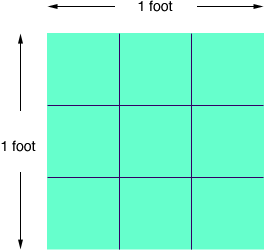Quandaries and Queries I am putting up backsplash tile in my kitchen and I need to know how many tiles I would need to use for about 45 square feet using 4x4 tiles.   Thank you Jeannette Hi Jeannette, The tiles are 4 inches on a side and there are 12 inches in a foot, so it takes 3 tiles to give a foot length and hence 3 × 3 = 9 tiles to make a square foot.You need to cover 45 square feet so you need 945 = 405 tiles. This calculation does not take wastage into account. The exact number will depend on the shape of the backsplash as well as the square footage. Penny Go to Math Central